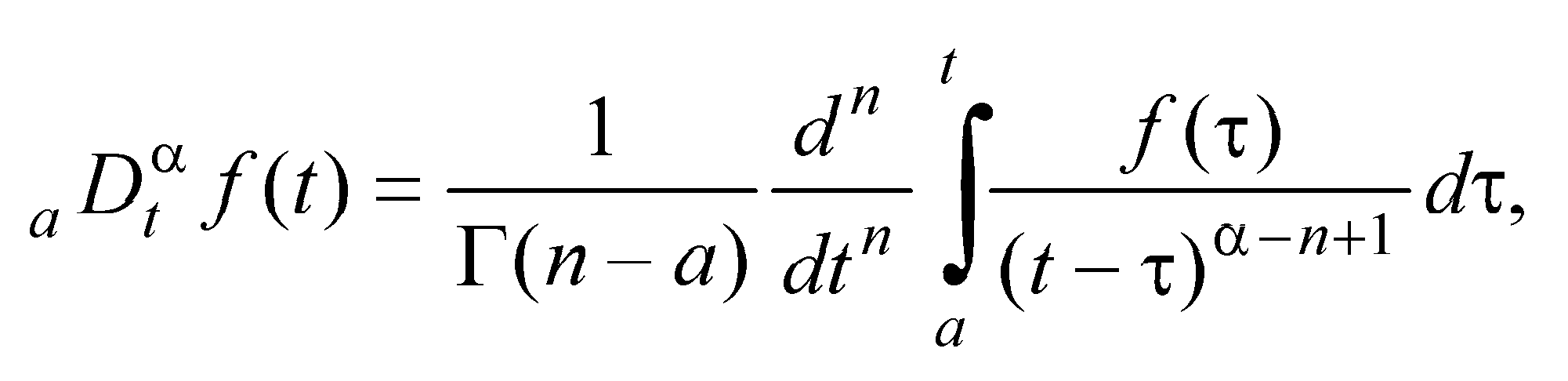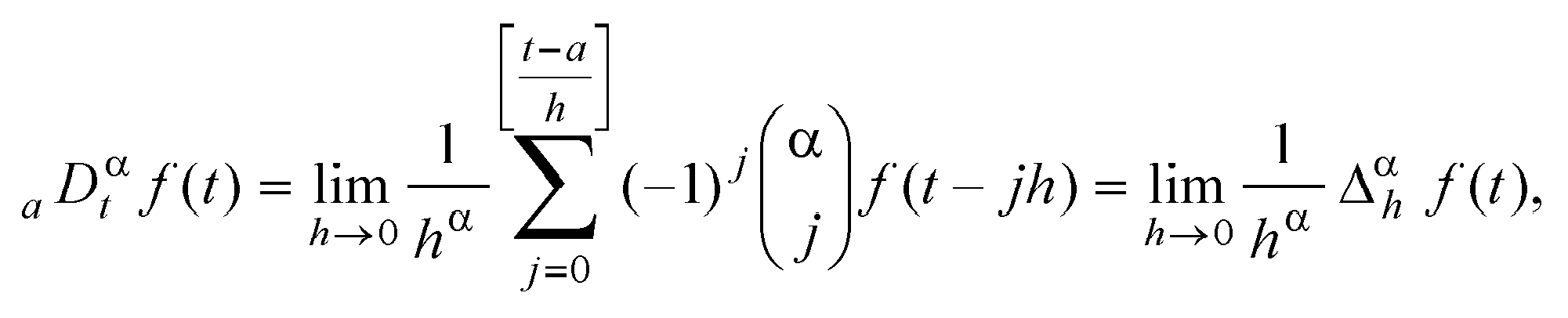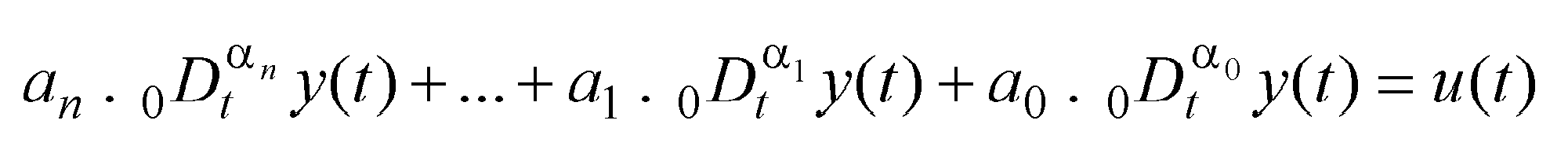# Fractional Calculus

## (differentiation and integration of arbitrary non-integer order)

1. Introduction

The term fractional calculus is no new. It is a generalization of the ordinary differentiation and integration to non-integer (arbitrary) order. The subject is as old as the calculus of differentiation and goes back to times when Leibniz, Gauss, and Newton invented this kind of calculation. In a letter to L`Hospital in 1695 Leibniz raised the following question: "Can the meaning of derivatives with integer order be generalized to derivatives with non-integer orders?" The story goes that L`Hospital was somewhat curious about that question and replied by another question to Leibniz. "What if the order will be 1/2?" Leibniz in a letter dated September 30, 1695 replied: "Il y a de l'apparence qu'on tirera un jour des consequences bien utiles de ces paradoxes, car il n'y a gueres de paradoxes sans utilité." The translation reads: "It will lead to a paradox, from which one day useful consequences will be drawn." The question raised by Leibniz for a fractional derivative was an ongoing topic in the last 300 years. Several mathematicians contributed to this subject over the years. People like Liouville, Riemann, and Weyl made major contributions to the theory of fractional calculus. The story on the fractional calculus continued with contributions from Fourier, Abel, Lacroix, Leibniz, Grunwald and Letnikov. For a historical survey the reader may consult the books of Oldham and Spanier or Miller and Ross. The historical developments culminated in two calculi which are based on the work of Riemann and Liouville (RL) at the one side and on the work of Grunwald and Letnikov (GL) at the other hand. Both formulations are connected. In the following section we will introduce first the RL calculus and then GL calculus.

2. Definition of fractional derivatives and integrals

The Riemann-Liouville definition is given asfor (n - 1 < a < n) and where Gama(.) is the well known Euler's gamma function. The Grunwald-Letnikov definition is given herewhere [ x ] means the integer part of x and h is time step. For a wide class of functions which appear in real physical and engineering applications, the Riemann-Liouville and the Grunwald-Letnikov definitions are equivalent.

3. Fractional differential equations

Fractional differential equations can be expressed in general formwhere ak, (k = 0, 1, 2, ..., n) are generally real numbers, an > ... >a1 > a0 >0 and ak, (k = 0, 1, 2, ..., n) are arbitrary constants.

4. Survey of applications

There are many fields of applications where we can use the fractional calculus, as for examples:

• Viscoelasticity,
• Control theory,
• Heat conduction,
• Electricity,
• Mechanics,
• Chaos and Fractals, etc.
References
1. Spanier, J. and Oldham, K. B. The Fractional Calculus: Integrations and Differentiations of Arbitrary Order. New York: Academic Press, 1974.
2. Nishimoto, K. Fractional Calculus. New Haven, CT: University of New Haven Press, 1989.
3. Samko, S. G.; Kilbas, A. A.; and Marichev, O. I. Fractional Integrals and Derivatives. Yverdon, Switzerland: Gordon and Breach, 1993.
4. Oustaloup, A. La derivation non entiere. Hermes, Paris, 1995.
5. Podlubny, I. Fractional Differential Equations. Academic Press, San Diego, 1999.

[Back to previous page]